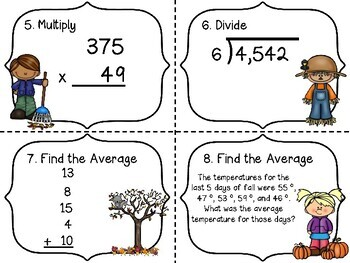# Finding Averages & More Fall Math Task Cards (Multiply, Divide, & Averages)Subject
Resource Type
File Type

PDF

(2 MB|16 pages)
Product Rating
Standards
• Product Description
• StandardsNEW

This great set of task cards includes practice for students in multiple digit multiplication and double digit multiplication, long division, and finding averages. Two different versions of finding averages are included: digits given and story problems with finding averages. 24 color Fall themed task cards are included as well as a color saving white background set of task cards. A student recording sheet as well as a teacher answer key is included. This set is perfect for individual practice, small group work, or Math centers.

Similar Math task card sets you maybe interested in include:

Multi-digit Subtraction Task Cards w/ regrouping & subtracting across zeros

Long Division w/ Remainders Task Cards

Solve multistep word problems posed with whole numbers and having whole-number answers using the four operations, including problems in which remainders must be interpreted. Represent these problems using equations with a letter standing for the unknown quantity. Assess the reasonableness of answers using mental computation and estimation strategies including rounding.
Multiply or divide to solve word problems involving multiplicative comparison, e.g., by using drawings and equations with a symbol for the unknown number to represent the problem, distinguishing multiplicative comparison from additive comparison.
Find whole-number quotients and remainders with up to four-digit dividends and one-digit divisors, using strategies based on place value, the properties of operations, and/or the relationship between multiplication and division. Illustrate and explain the calculation by using equations, rectangular arrays, and/or area models.
Multiply a whole number of up to four digits by a one-digit whole number, and multiply two two-digit numbers, using strategies based on place value and the properties of operations. Illustrate and explain the calculation by using equations, rectangular arrays, and/or area models.
Total Pages
16 pages
N/A
Teaching Duration
N/A
Report this Resource to TpT
Reported resources will be reviewed by our team. Report this resource to let us know if this resource violates TpT’s content guidelines.